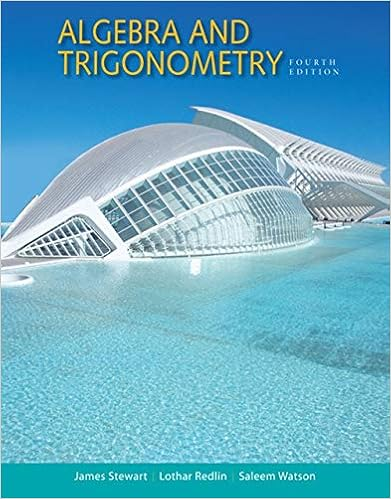# Use this figure for the following questions 12 points

• Test Prep
• 10
• 100% (19) 19 out of 19 people found this document helpful

This preview shows page 5 - 7 out of 10 pages.

##### We have textbook solutions for you!
The document you are viewing contains questions related to this textbook.The document you are viewing contains questions related to this textbook.
Chapter 14 / Exercise 54
Algebra and Trigonometry
Redlin/StewartExpert Verified
16. Use this figure for the following questions. (12 points total for this question) A. What does this figure illustrate? How does this work with the sickle cell mutation in the human hemoglobin gene? (4 points)
B. What will be the frequencies of the A 1 and A 2 alleles at equilibrium (show your work)? (4 points)
C. Explain what would happen if the frequency of the A 1 allele were to increase above its equilibrium value. What kind of selection does this illustrate, and what kind of equilibrium does it lead to? (4 points)
##### We have textbook solutions for you!
The document you are viewing contains questions related to this textbook.The document you are viewing contains questions related to this textbook.
Chapter 14 / Exercise 54
Algebra and Trigonometry
Redlin/StewartExpert Verified
NAME ________________________ EID _______________ 17. Use this figure for the following questions. (7 points total for this question) A. What does this figure illustrate, and how does that work? (3 points)
B. If A 1 A 1 and A 1 A 2 individuals exhibit phenotype P 1 , and A 2 A 2 individuals exhibit phenotype P 2 , what will happen to the two phenotypes and the two alleles if the P phenotype increases in frequency to above its equilibrium value? (4 points) 2
6
•••